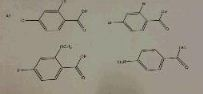# a. rank the following in order of most acidic to least acidic. b. list 5.ways to make carboxylic...

## Question:

a. rank the following in order of most acidic to least acidic. b. list 5.ways to make carboxylic acids.## Preparation of Carboxylic Acid:

Carboxylic acids are organic compounds with a carboxylic functional group that has a carbonyl and a hydroxyl functional group.

It has certain functional derivatives like ester, amide, acid halide, and acid anhydride.

The carboxylic acid and its derivatives can be interconverted using certain reagents.

• The image is so blurred that the compounds cannot be seen.
• Following are the five ways to prepare carboxylic acid:

(1) {eq}\rm CH_{3}COOCH_{3} + H_{2}O \rightarrow CH_{3}COOH + CH_{3}OH {/eq}

(2) {eq}CH_{3}COCl + H_{2}O \rightarrow CH_{3}COOH + HCl {/eq}

(3) {eq}CH_{3}COOCOCH_{3} + H_{2}O \rightarrow 2CH_{3}COOH {/eq}

(4) {eq}CH_{3}CONH_{2} + H_{2}O \rightarrow CH_{3}COOH + NH_{3} {/eq}

(5) {eq}R-OH + KMnO_{4} \rightarrow R-COOH {/eq}Carboxylic Acid: Structural Formula, Properties & Uses

from AP Chemistry: Tutoring Solution

Chapter 15 / Lesson 15
73K

Support